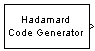Generate Hadamard code from orthogonal set of codes

## Library

Sequence Generators sublibrary of Comm Sources

•## Description

The Hadamard Code Generator block generates a Hadamard code from a Hadamard matrix, whose rows form an orthogonal set of codes. Orthogonal codes can be used for spreading in communication systems in which the receiver is perfectly synchronized with the transmitter. In these systems, the despreading operation is ideal, as the codes are decorrelated completely.

The Hadamard codes are the individual rows of a Hadamard matrix. Hadamard matrices are square matrices whose entries are +1 or -1, and whose rows and columns are mutually orthogonal. If N is a nonnegative power of 2, theN-by-N Hadamard matrix, denoted HN, is defined recursively as follows.

`$\begin{array}{c}{H}_{1}=\left[1\right]\\ {H}_{2N}=\left[\begin{array}{cc}{H}_{N}& {H}_{N}\\ {H}_{N}& -{H}_{N}\end{array}\right]\end{array}$`

The N-by-N Hadamard matrix has the property that

HNHNT = NIN

where IN is the N-by-N identity matrix.

The Hadamard Code Generator block outputs a row of HN. The output is bipolar. You specify the length of the code, N,by the Code length parameter. The Code length must be a power of 2. You specify the index of the row of the Hadamard matrix, which is an integer in the range [0, 1, ... , N-1], by the Code index parameter.

## Parameters

Code length

A positive integer that is a power of two specifying the length of the Hadamard code.

Code index

An integer between 0 and N-1, where N is the Code length, specifying a row of the Hadamard matrix.

Sample time

Output sample time, specified as `-1` or a positive scalar that represents the time between each sample of the output signal. If Sample time is set to `-1`, the sample time is inherited from downstream. For information on the relationship between Sample time and Samples per frame, see Sample Timing.

Samples per frame

Samples per frame, specified as a positive integer indicating the number of samples per frame in one channel of the output data. For information on the relationship between Sample time and Samples per frame, see Sample Timing.

Output data type

The output type of the block can be specified as an `int8` or `double`. By default, the block sets this to `double`.

Simulate using

Select the simulation mode.

`Code generation`

On the first model run, simulate and generate code. If the structure of the block does not change, subsequent model runs do not regenerate the code.

If the simulation mode is ```Code generation```, System objects corresponding to the blocks accept a maximum of nine inputs.

`Interpreted execution`

Simulate model without generating code. This option results in faster start times but can slow subsequent simulation performance.

## Examples

### Orthogonal Spreading - Single-User vs. Two-User Comparison

This example model compares a single-user system vs. a two-user data transmission system with the two data streams being independently spread by different orthogonal codes.

The model uses random binary data which is BPSK modulated (real), spread by Hadamard codes of length 64 and then transmitted over an AWGN channel. The receiver consists of a despreader followed by a BPSK demodulator. Open the model here: hadamard_block_example1.

```modelname = 'hadamard_block_example1'; open_system(modelname); sim(modelname);```For the same data and channel settings, the model calculates the performance for one- and two-user transmissions.

Note that for the individual users, the error rates are exactly the same in both cases. This shows that perfect despreading is possible due to the ideal cross-correlation properties of the Hadamard codes.

To experiment with this model further, specify a different Code length or Code index for the individual users to examine the variations in relative performance.

`close_system(modelname, 0);`

### Orthogonal Spreading - Multipath Scenario

This example model considers a single-user system in which the signal is transmitted over multiple paths. This is similar to a mobile channel environment where the signals are received over multiple paths, each of which have different amplitudes and delays. To take advantage of the multipath transmission, the receiver employs diversity reception which combines the independent paths coherently.

Note, to keep the system simple, no shadowing effects are considered and the receiver has a priori knowledge of the number of paths and their respective delays. Open the model here: hadamard_block_example2.

```modelname = 'hadamard_block_example2'; open_system(modelname); sim(modelname);```For the data transmission with the same spreading code that was used in the first example, we now see deterioration in performance when compared with that example (compare the 180 errors with 81 in the previous case). This can be attributed to the non-ideal auto-correlation values of the orthogonal spreading codes chosen, which prevents perfect resolution of the individual paths. Consequently, we don't see the merits of diversity combining.

To experiment with this model further, try selecting other path delays to see how the performance varies for the same code. Also try different codes with the same delays.

`close_system(modelname, 0);`

expand all

## Compatibility Considerations

expand all

Behavior changed in R2020a